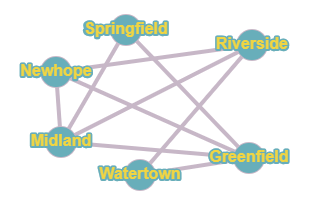# Transportation An "X" in the table below indicates a direct train route between the corresponding cities. Draw a graph that represents this information, in which each vertex represents a city and an edge connects two vertices if there is a train route between the corresponding cities.### Mathematical Excursions (MindTap C...

4th Edition
Richard N. Aufmann + 3 others
Publisher: Cengage Learning
ISBN: 9781305965584

#### Solutions

Chapter
Section### Mathematical Excursions (MindTap C...

4th Edition
Richard N. Aufmann + 3 others
Publisher: Cengage Learning
ISBN: 9781305965584
Chapter 5.1, Problem 1ES
Textbook Problem
43 views

## Transportation An "X" in the table below indicates a direct train route between the corresponding cities. Draw a graph that represents this information, in which each vertex represents a city and an edge connects two vertices if there is a train route between the corresponding cities.To determine

To graph:

To graph the information representing the routes between cities.

### Explanation of Solution

Given information:

Given, an "X" in the given table indicates a direct train route between the corresponding cities.

A graph is to be made where each vertex represents a city and an edge connects two vertices if there is a train route between the corresponding cities.

Calculation:

Given, there are six cities...

### Still sussing out bartleby?

Check out a sample textbook solution.

See a sample solution

#### The Solution to Your Study Problems

Bartleby provides explanations to thousands of textbook problems written by our experts, many with advanced degrees!

Get Started

Find more solutions based on key concepts
Let y = x2 + 2. a. Find the average rate of change of y with respect to x over the intervals [1, 2], [1, 1.5], ...

Applied Calculus for the Managerial, Life, and Social Sciences: A Brief Approach

The equation of the line through (4, 10, 8) and (3, 5, 1) is:

Study Guide for Stewart's Multivariable Calculus, 8th

In Problems 2326 first use (20) to express the general solution of the given differential equation in terms of ...

A First Course in Differential Equations with Modeling Applications (MindTap Course List)

12. The Powerball lottery is played twice each week in 31 states, the District of Columbia, and the Virgin Isla...

Modern Business Statistics with Microsoft Office Excel (with XLSTAT Education Edition Printed Access Card) (MindTap Course List)

Reminder Round all the answers to two decimal places unless otherwise indicated. A Leaking Can The side of a cy...

Functions and Change: A Modeling Approach to College Algebra (MindTap Course List)